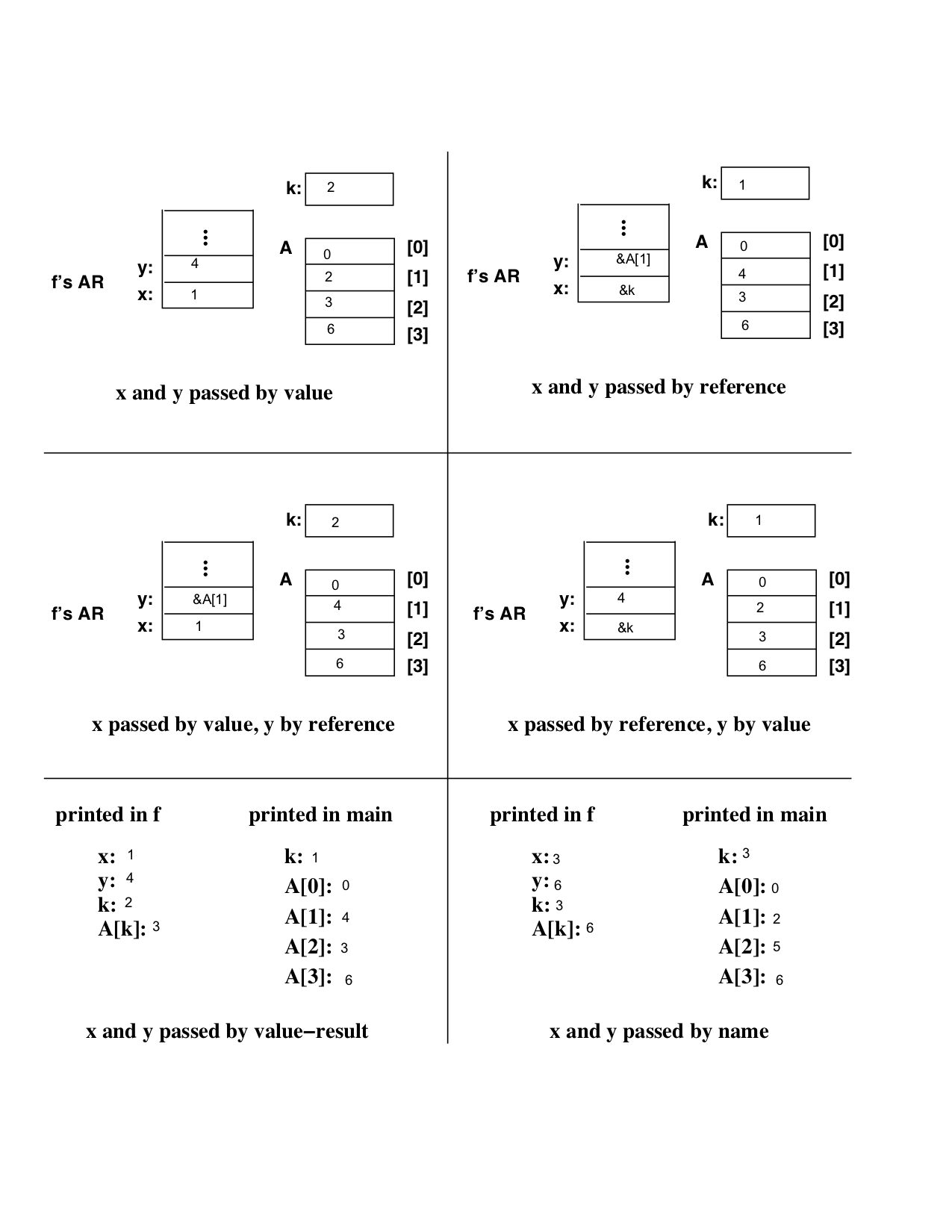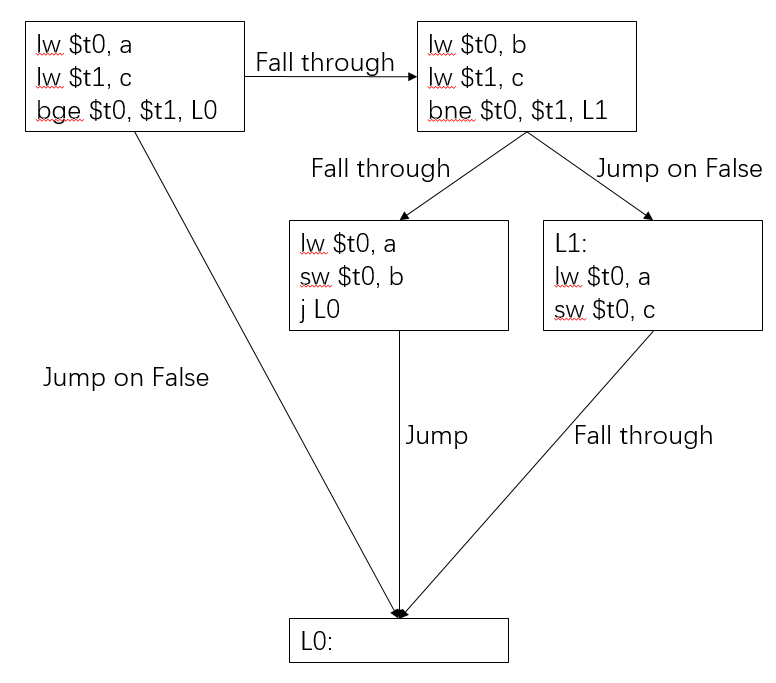# CS536 Homework 5 Solution

## Question 1:## Question 2:## Question 3:

### Part a:

Yes. The expression can be evaluated by the above approach

### Part b:

The expression can be evaluated using at least 3 registers:

expression AST pseudo code
` (a - b) - (c - d) `
```      -
/   \
-     -
/ \   / \
a   b c   d
```
```  load a into R0
R0 = R0 - R1
R1 = R1 - R2
R0 = R0 - R1
```

### Part c:

The expression can be evaluated using at least 4 registers:

expression AST pseudo code
` ((a - b) - (c - d)) - ((e - f) - (g - h)) `
```            -
/     \
/       \
/         \
-           -
/   \       /   \
-     -     -     -
/ \   / \   / \   / \
a   b c   d e   f g   h
```
```  load a into R0
R0 = R0 - R1